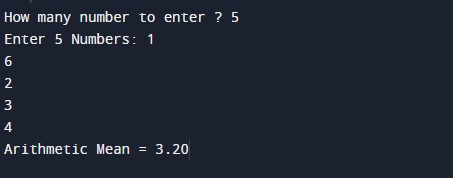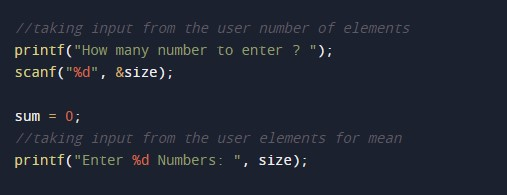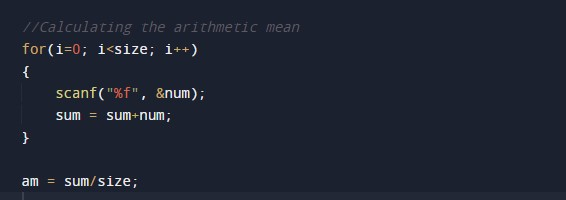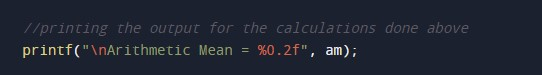C Program to Calculate Arithmetic Mean

In this tutorial you will learn about the C Program to Calculate Arithmetic Mean and its application with practical example.

C Program to Calculate Arithmetic Mean

In this tutorial, we will learn to create a C program that will Calculate Arithmetic Mean using C programming.

Prerequisites

Before starting with this tutorial we assume that you are best aware of the following C programming topics:

• Operators in C Programming.
• Basic Input and Output function in C Programming.
• Basic C programming.
• Arithmetic operations in C Programming.
• For Loop in C programming.

Program to Calculate Arithmetic Mean

In c programming, it is possible to take numerical input from the user and Calculate the Arithmetic Mean with the help of a very small amount of code. The C language has many types of header libraries which has supported function in them. With the help of these functions, the programming is easy.

With the help of this program, we can Calculate Arithmetic Mean.

Here,

We will first take the input

The second will make Calculate the Arithmetic Mean.

Output:-In the above program, we have first initialized the required variable.• size = it will hold the integer value.
• i = it will hold the integer value.
• num = it will hold the float value.
• sum = it will hold the float value.
• am = it will hold the float value.

Input number from the user.Program Logic Code.Printing the output.In this tutorial we have learn about the C Program to Calculate Arithmetic Mean and its application with practical example. I hope you will like this tutorial.